# 基于Lagrange约束因子修正迭代初值的TDOA定位算法TDOA Localization Algorithm Based on Lagrange Constraint Factor to Modify the Initial Value of Iteration

DOI: 10.12677/AAM.2020.93045, PDF, HTML, XML, 下载: 122  浏览: 216

Abstract: For the TDOA positioning method, different algorithms determine different positioning accuracy, and the traditional Taylor series expansion method has a strong dependence on the suitability of the initial value selection. In order to improve the positioning accuracy, this paper proposes a method to correct the initial position error by introducing the Lagrange constraint factor on the basis of Taylor-series estimation. Simulation experiment I shows that under the same simulation conditions, the optimal positioning accuracy of the traditional Taylor series expansion method is lower than that of the Chan algorithm. Simulation experiment II shows that when the number of satellite observation platform networks is 4 and 6 respectively, the positioning accuracy of the modified algorithm proposed in this paper is better than the traditional Chan algorithm and Taylor algorithm.

1. 引言

TDOA定位方程的解算方式与定位精度有直接关系 。最早的定位算法是1976年Foy等人提出的Taylor级数展开法 ，本质上是一种迭代算法，类似的，还有利用Newton迭代法进行位置求解，迭代算法的优点是定位精度高 ，但是缺点迭代算法计算复杂，且需要选取合适的目标位置初始值，因此如何优化上述算法十分有研究意义。除了迭代法，还有以Y.T. Chan提出的Chan算法  为代表的解析法。此外，还有一种闭式解法思想，代表算法有球面相交法(SX)  、平面相交法(PX)  和球面插值法(SI) ，尽管这些算法都可以求得目标的位置解，但是闭式解法有一个共同的缺点，定位性能无法达到克拉美罗下界 。

2. TDOA定位原理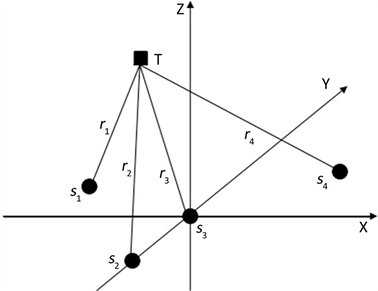Figure 1. Schematic diagram of 3D TDOA positioning principle

$\left\{\begin{array}{l}{r}_{1}^{2}={\left(x-{x}_{1}\right)}^{2}+{\left(y-{y}_{1}\right)}^{2}+{\left(z-{z}_{1}\right)}^{2}\hfill \\ {r}_{2}^{2}={\left(x-{x}_{2}\right)}^{2}+{\left(y-{y}_{2}\right)}^{2}+{\left(z-{z}_{2}\right)}^{2}\hfill \\ {r}_{3}^{2}={\left(x-{x}_{3}\right)}^{2}+{\left(y-{y}_{3}\right)}^{2}+{\left(z-{z}_{3}\right)}^{2}\hfill \\ {r}_{4}^{2}={\left(x-{x}_{4}\right)}^{2}+{\left(y-{y}_{4}\right)}^{2}+{\left(z-{z}_{4}\right)}^{2}\hfill \end{array}$ (1)

$\left\{\begin{array}{l}\Delta {r}_{1}={r}_{2}-{r}_{1}=c\Delta {t}_{1}\hfill \\ \Delta {r}_{2}={r}_{3}-{r}_{1}=c\Delta {t}_{2}\hfill \\ \Delta {r}_{3}={r}_{4}-{r}_{1}=c\Delta {t}_{3}\hfill \end{array}$ (2)

$\left\{\begin{array}{l}\left({x}_{1}-{x}_{2}\right)x+\left({y}_{1}-{y}_{2}\right)y+\left({z}_{1}-{z}_{2}\right)z={k}_{1}+{r}_{1}\Delta {r}_{1}\hfill \\ \left({x}_{1}-{x}_{3}\right)x+\left({y}_{1}-{y}_{3}\right)y+\left({z}_{1}-{z}_{3}\right)z={k}_{2}+{r}_{2}\Delta {r}_{2}\hfill \\ \left({x}_{1}-{x}_{4}\right)x+\left({y}_{1}-{y}_{4}\right)y+\left({z}_{1}-{z}_{4}\right)z={k}_{3}+{r}_{3}\Delta {r}_{3}\hfill \end{array}$ (3)

3. Chan算法

1994年学者Y.T. Chan提出了一种本质并非迭代法的非线性方程求解算法，该算法本质是解析式法，并且不需要设置初值，计算量也大大降低，于是该算法被命名为Chan算法。

$AX=F$ (4)

$\stackrel{^}{X}={\left({A}^{\text{T}}A\right)}^{-1}{A}^{\text{T}}F$ (5)

${\left({A}^{\text{T}}A\right)}^{-1}{A}^{\text{T}}={\left[{a}_{ij}\right]}_{3×3}=\left[\begin{array}{ccc}{a}_{11}& {a}_{12}& {a}_{13}\\ {a}_{21}& {a}_{22}& {a}_{23}\\ {a}_{31}& {a}_{31}& {a}_{33}\end{array}\right]$ (6)

$\stackrel{^}{X}=\left[\begin{array}{c}\stackrel{^}{x}\\ \stackrel{^}{y}\\ \stackrel{^}{z}\end{array}\right]=\left[\begin{array}{c}{m}_{1}+{n}_{1}{r}_{1}\\ {m}_{2}+{n}_{2}{r}_{2}\\ {m}_{3}+{n}_{3}{r}_{3}\end{array}\right]$ (7)

$a{r}_{1}{}^{2}+2b{r}_{1}+c=0$ (8)

1) 当判别式 $\Delta >0$ 时，式(11)有两解，此时出现了时差定位模糊现象；

2) 当判别式 $\Delta =0$ 时，式(11)有一解，此时的解便是辐射源目标的位置解，没有出现时差定位模糊现象；

3) 当判别式 $\Delta <0$ 时，式(11)无解，时差定位曲线无交点，定位失效。

${r}_{1}=\frac{-b±\sqrt{{b}^{2}-ac}}{a}$ (9)

4. Taylor级数展开法

Taylor级数展开法本质上是一种迭代算法，核心思想  是：将TDOA定位方程按照Taylor级数终值定理展开，并忽略二阶及二阶以上的分量，在开始迭代前为目标位置赋予初值，之后在此基础上进行迭代，在每一次的迭代过程中通过局部最小二乘解(Least Squares, LS)逐渐收敛于辐射源目标位置解。

1) 根据TDOA定位原理，将迭代初始值设为 ${X}^{\prime }={\left({x}^{\prime },{y}^{\prime },{z}^{\prime }\right)}^{\text{T}}$，与真实位置的测量误差记为 $\delta ={\left(\Delta x,\Delta y,\Delta y\right)}^{\text{T}}$，则有如下关系：

$X={X}^{\prime }+\delta$ (10)

2) 在迭代初始值处按照Taylor级数展开，去除二阶以上高阶分量的同时仅保留一阶分量，得到如下表达式：

$e\approx H-G\delta$ (11)

$G=\left[\begin{array}{ccc}{\frac{\partial \Delta {r}_{2}}{\delta {x}^{\prime }}|}_{\left({x}^{\prime },{y}^{\prime },{z}^{\prime }\right)}& {\frac{\partial \Delta {r}_{2}}{\delta {y}^{\prime }}|}_{\left({x}^{\prime },{y}^{\prime },{z}^{\prime }\right)}& {\frac{\partial \Delta {r}_{2}}{\delta {z}^{\prime }}|}_{\left({x}^{\prime },{y}^{\prime },{z}^{\prime }\right)}\\ {\frac{\partial \Delta {r}_{3}}{\delta {x}^{\prime }}|}_{\left({x}^{\prime },{y}^{\prime },{z}^{\prime }\right)}& {\frac{\partial \Delta {r}_{3}}{\delta {y}^{\prime }}|}_{\left({x}^{\prime },{y}^{\prime },{z}^{\prime }\right)}& {\frac{\partial \Delta {r}_{3}}{\delta {z}^{\prime }}|}_{\left({x}^{\prime },{y}^{\prime },{z}^{\prime }\right)}\\ {\frac{\partial \Delta {r}_{4}}{\delta {x}^{\prime }}|}_{\left({x}^{\prime },{y}^{\prime },{z}^{\prime }\right)}& {\frac{\partial \Delta {r}_{4}}{\delta {y}^{\prime }}|}_{\left({x}^{\prime },{y}^{\prime },{z}^{\prime }\right)}& {\frac{\partial \Delta {r}_{4}}{\delta {z}^{\prime }}|}_{\left({x}^{\prime },{y}^{\prime },{z}^{\prime }\right)}\end{array}\right]=\left[\begin{array}{ccc}\frac{{x}_{1}-{x}^{\prime }}{{r}_{1}}-\frac{{x}_{2}-{x}^{\prime }}{{r}_{2}}& \frac{{y}_{1}-{y}^{\prime }}{{r}_{1}}-\frac{{y}_{2}-{x}^{\prime }}{{r}_{2}}& \frac{{z}_{1}-{z}^{\prime }}{{r}_{1}}-\frac{{z}_{2}-{z}^{\prime }}{{r}_{2}}\\ \frac{{x}_{1}-{x}^{\prime }}{{r}_{1}}-\frac{{x}_{3}-{x}^{\prime }}{{r}_{3}}& \frac{{y}_{1}-{y}^{\prime }}{{r}_{1}}-\frac{{y}_{3}-{x}^{\prime }}{{r}_{3}}& \frac{{z}_{1}-{z}^{\prime }}{{r}_{1}}-\frac{{z}_{3}-{z}^{\prime }}{{r}_{3}}\\ \frac{{x}_{1}-{x}^{\prime }}{{r}_{1}}-\frac{{x}_{4}-{x}^{\prime }}{{r}_{4}}& \frac{{y}_{1}-{y}^{\prime }}{{r}_{1}}-\frac{{y}_{4}-{x}^{\prime }}{{r}_{4}}& \frac{{z}_{1}-{z}^{\prime }}{{r}_{1}}-\frac{{z}_{4}-{z}^{\prime }}{{r}_{4}}\end{array}\right].$

3) 用加权最小二乘法得出初始位置处的最大似然估计：

$\delta ={\left[\begin{array}{ccc}\Delta x& \Delta y& \Delta z\end{array}\right]}^{\text{T}}={\left({G}^{-1}{Q}^{-1}G\right)}^{-1}{G}^{\text{T}}{Q}^{-1}H$ (12)

4) 设定一个门限精度 ${\epsilon }_{t}$

$\left\{\begin{array}{l}\stackrel{^}{x}={x}^{\prime }+\Delta x\hfill \\ \stackrel{^}{y}={y}^{\prime }+\Delta y\hfill \\ \stackrel{^}{z}={z}^{\prime }+\Delta z\hfill \end{array}$ (13)

5) 用最新得到估计值的估计值替代上一个估计值，并重复步骤3)和步骤4)。

5. 基于Lagrange约束因子的修正算法

Chan算法计算简单但是有时差模糊现象，该现象严重可导致系统定位功能失效；Taylor算法尽管计算复杂，但每次都能得到确定的位置解，此外初值选取的合适与否对定位结果有直接的影响。随着计算机计算速度的加快，复杂的计算已经变得不再复杂，为此提出一种基于Lagrange约束因子的解算方法。

$AX=b$ (14)

$X={\left[\begin{array}{cccc}x& y& z& {r}_{1}\end{array}\right]}^{\text{T}}$

$b={\left[\begin{array}{ccc}{k}_{1}& {k}_{2}& {k}_{3}\end{array}\right]}^{\text{T}}$

$\stackrel{^}{X}={\left({A}^{\text{T}}A-{\sigma }^{2}I\right)}^{-1}{A}^{\text{T}}b=X+\Delta X$ (15)

${r}_{1}^{2}={x}^{2}+{y}^{2}+{z}^{2}$ (16)

${X}^{\text{T}}gX=0$ (17)

$L\left(X,\lambda \right)={\left(AX-b\right)}^{\text{T}}\left(AX-b\right)+\lambda {X}^{\text{T}}gX$ (18)

$L\left(X,\lambda \right)$ 求一阶偏导数并令其为零：

$\frac{\partial L\left(X,\lambda \right)}{\partial X}={\left({A}^{\text{T}}A+\lambda g\right)}^{-1}-{A}^{\text{T}}b=0$ (19)

$X={\left({A}^{\text{T}}A+\lambda g\right)}^{-1}{A}^{\text{T}}b$ (20)(21)

${\left({A}^{\text{T}}A+\lambda g\right)}^{-1}=U{\left(I+\lambda \Lambda \right)}^{-1}{U}^{-1}{\left({A}^{\text{T}}A\right)}^{-1}$ (22)

${E}^{\text{T}}{\left(I+\lambda \Lambda \right)}^{-1}\Lambda {\left(I+\lambda \Lambda \right)}^{-1}h=0$ (23)

$\underset{i=1}{\overset{4}{\sum }}\frac{{e}_{i}{\lambda }_{i}{h}_{i}}{{\left(\lambda {\lambda }_{i}+1\right)}^{2}}=0$ (24)

$\Delta X={\left({A}^{\text{T}}A-{\sigma }^{2}I\right)}^{-1}{A}^{\text{T}}b+\lambda \left({A}^{\text{T}}A\right)gX-{A}^{-1}b$ (25)

$\stackrel{^}{X}={\left({A}^{\text{T}}A-{\sigma }^{2}I\right)}^{-1}{A}^{\text{T}}b+\left[\lambda \left({A}^{\text{T}}A\right)g+I\right]X-{A}^{-1}b$ (26)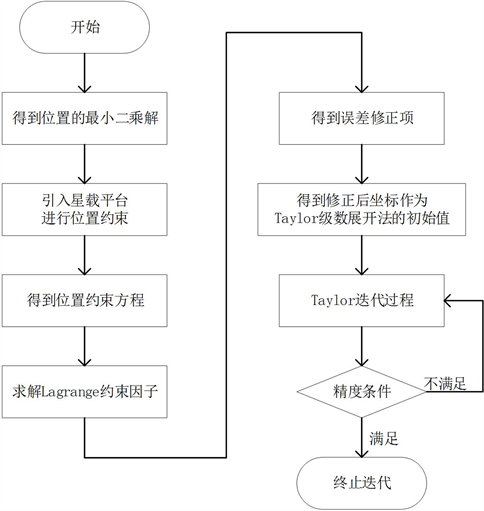Figure 2. The principle diagram of Lagrange constraint factor correction algorithm

6. 实验结果及分析

$RMSE\left(X\right)=\sqrt{E\left[{‖X-\stackrel{^}{X}‖}_{2}^{2}\right]}=\sqrt{\underset{i=1}{\overset{{T}_{s}}{\sum }}{\left({X}_{i}-\stackrel{^}{X}\right)}^{2}/{T}_{s}}$ (27)Table 1. Location distribution of space borne observation platform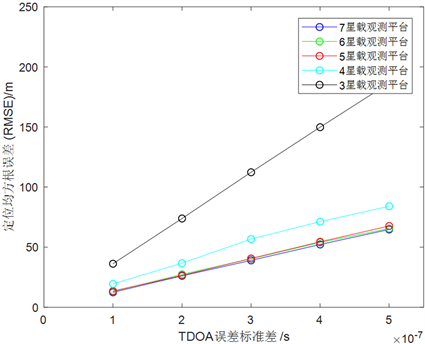Figure 3. Performance of Chan algorithm under different spaceborne observation platforms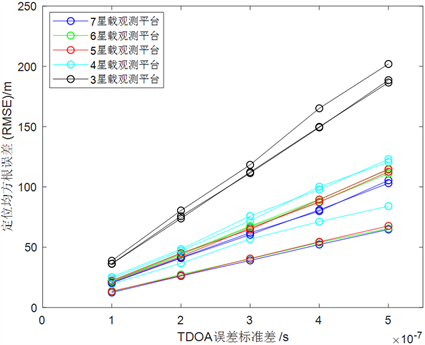Figure 4. Performance of Taylor algorithm under different spaceborne observation platforms

$MSE\left(X\right)=E\left[{‖X-\stackrel{^}{X}‖}_{2}^{2}\right]=\underset{i=1}{\overset{{T}_{s}}{\sum }}{\left({X}_{i}-\stackrel{^}{X}\right)}^{2}/{T}_{s}$ (28)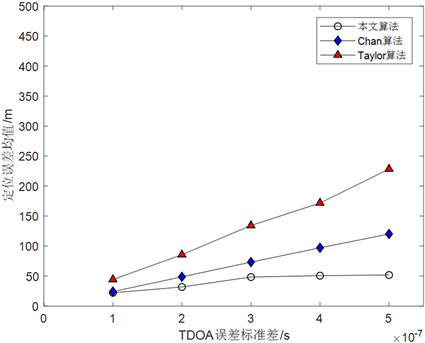Figure 5. Performance comparison of three positioning algorithms under four spaceborne observation platforms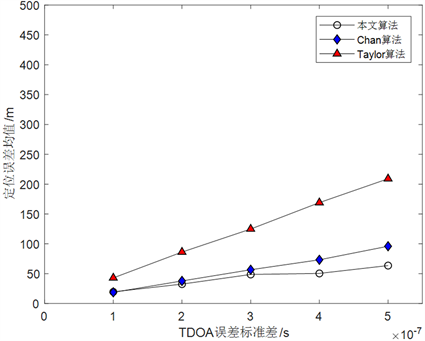Figure 6. Performance comparison of three positioning algorithms under six spaceborne observation platforms

7. 结论

  Yang, X., Li, J. and Zhang, S. (2014) Ionospheric Correction for Spaceborne Single-Frequency GPS Based on Single Layer Model. Journal of Earth System Science, 123, 767-778. https://doi.org/10.1007/s12040-014-0442-z  Ankit, J., Pascal, P., Rolland, F., et al. (2018) Accurate Time Difference of Arrival Estimation for HF Radio Broadcast Signals. Microwave and Optical Technology Letters, 60, 1406-1410. https://doi.org/10.1002/mop.31178  Adamy, D. (2005) Precision Emitter Location by FDOA. The Journal of Electronic Defense, 28, 62-63.  刁鸣, 王越. 基于多普勒频率变化率的无源定位算法研究[J]. 系统工程与电子技术, 2006, 28(5): 696-698.  Boccadoro, M., De, A. and Valigi, P. (2012) TDOA Positioning in NLOS Scenarios by Particle Filtering. Wireless Networks, 18, 579-589. https://doi.org/10.1007/s11276-012-0420-9  Foy, W.H. (1976) Position-Location Solution by Taylor-Series Estimation. IEEE Transactions on Aerospace and Electronic Systems, 12, 187-194. https://doi.org/10.1109/TAES.1976.308294  Chernoguz, N. (1995) A Smoothed Newton-Gauss Method with Application to Bearing-Only Position Location. IEEE Transactions on Signal Processing, 43, 2011-2013. https://doi.org/10.1109/78.403366  Chan, Y., So, H., Lee, B., et al. (2013) Angle of Arrival Localization of an Emitter from Air Platforms. IEEE Canadian Conference of Electrical and Computer Engineering, Regina, 5-8 May 2013, 1-5. https://doi.org/10.1109/CCECE.2013.6567733  Anna, O. and Alon, A. (2012) An Extended Spheri-cal-Intersection Method for Acoustic Sensor Network Localization with Unknown Propagation Speed. 2012 IEEE 27th Convention of Electrical and Electronics Engineers in Israel, Eilat, 14-17 November 2012.  Zhang, X., Wu, D., Liu, C., et al. (2012) Analysis on Capacity of Reformed Signalized Plane Intersection. 2012 2nd International Conference on Remote Sensing, Environment and Transportation Engineering, Nanjing, 1-3 June 2012. https://doi.org/10.1109/RSETE.2012.6260396  Freeden, W. and T?rnig, W. (2011) On Spherical Spline In-terpolation and Approximation. Mathematical Methods in the Applied Sciences, 3, 551-575. https://doi.org/10.1002/mma.1670030139  Yacine, A., Julio, C. and Hurd, T. (2009) Portfolio Choice with Jumps: A Closed-Form Solution. Annals of Applied Probability, 19, 556-584. https://doi.org/10.1214/08-AAP552  李莉, 邓平, 刘林. Taylor级数展开法定位及其性能分析[J]. 西南交通大学学报, 2002, 37(6): 684-688.  Annibalem, P., Filos, J. and Naylor, P. (2012) TDOA-Based Speed of Sound Estimation for Air Temperature and Roomgeometry Inference. IEEE Transactions on Audio, and Language Processing, 21, 234-246. https://doi.org/10.1109/TASL.2012.2217130